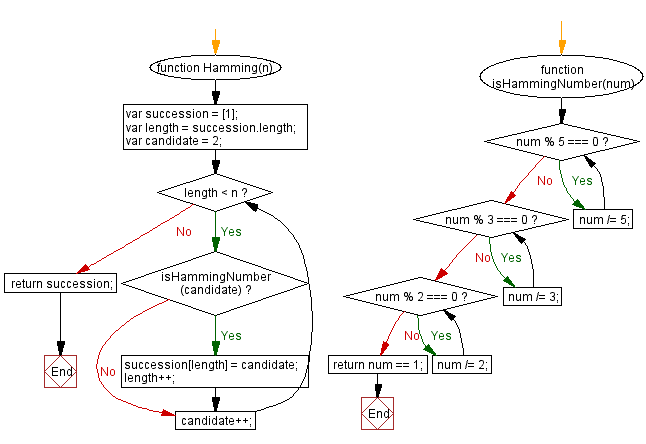# JavaScript: Show the Hamming numbers

## JavaScript Math: Exercise-44 with Solution

Write a JavaScript function to show the first twenty Hamming numbers. Hamming numbers are numbers with prime factors of 2, 3 and 5

Sample Solution:-

HTML Code:

``````<!DOCTYPE html>
<html>
<meta charset="utf-8">
<title>JavaScript function to show the Hamming numbers</title>
<body>

</body>
</html>
```
```

JavaScript Code:

``````function Hamming(n) {
var succession = ;
var length = succession.length;
var candidate = 2;
while (length < n) {
if (isHammingNumber(candidate)) {
succession[length] = candidate;
length++;
}
candidate++;
}
return succession;
}
function isHammingNumber(num) {
while (num % 5 === 0) num /= 5;
while (num % 3 === 0) num /= 3;
while (num % 2 === 0) num /= 2;

return num == 1;
}

console.log(Hamming(20));
```
```

Sample Output:

```[1,2,3,4,5,6,8,9,10,12,15,16,18,20,24,25,27,30,32,36]
```

Pictorial Presentation:Flowchart:Live Demo:

See the Pen javascript-math-exercise-44 by w3resource (@w3resource) on CodePen.

Improve this sample solution and post your code through Disqus

What is the difficulty level of this exercise?

Test your Programming skills with w3resource's quiz.

﻿